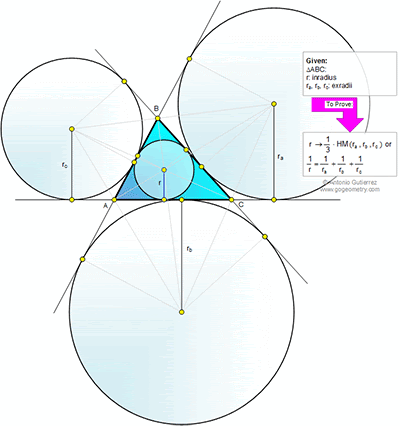## Thursday, June 14, 2012

Geometry Problem
Level: Mathematics Education, High School, Honors Geometry, College.

Click the figure below to see the complete problem 765.1.http://img43.imageshack.us/img43/9397/problem765.png

Denote S(XYZ)= area of triangle XYZ
Draw additional lines per attached sketch.
We have ∆AA’F similar to ∆AIE
So IE/A’F=r/rA =AI/AA’= S(IC’B’)/S(A’B’C’)
Similarly r/rB=S(IA’C’)/S(A’B’C’) and r/rC=S(IA’B’)/S(A’B’C’)
But S(A’B’C’)=S(IA’C’)+S(IA’B’)+S(IC’B’)
So r/rA+r/rB+r/rC= 1 or 1/r=1/rA+1/rB+1/rC

2.Let S be the area of the triangle and X = (1/2)(a+b+c), where a,b,c are the side lengths of the triangle.
By the properties of the exradii, S=(Ra)(X-a) ; S=(Rb)(X-b) ; S=(Rc)(X-c).
Therefore,
S/(Ra)=(X-a)
S/(Rb)=(X-b)
S/(Rc)=(X-c)
By summing up, S[(1/Ra)+(1/Rb)+(1/Rc)]=3X-(a+b+c) = X.
By the properties of the inradii, S=RX => X =S/R
So,
S/R = S[(1/Ra)+(1/Rb)+(1/Rc)]
1/R = (1/Ra)+(1/Rb)+(1/Rc)

q.e.d.

Now,

1.Thank you very much for that) I searched this answer all day))

3.Let BC touch incircle at X and excircle opp to B at Y
Triangles BIX and BI₂Y are similar
r/r₂ = AX/AY = (s - b)/s
Similarly r/r₃ = (s - c)/s and r/r₁= (s - a)/s
r/r₁ + r/r₂ + r/r₃
=(s - a)/s + (s - b)/s + (s - c)/s
= [3s - (a + b + c)] = (3s - 2s) /s = 1
Hence 1/r₁ + 1/r₂ + 1/r₃ = 1/r